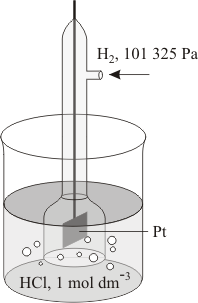A# electrode

An electrode is an electrical conductor, usually a wire or a rod, used to make contact with a non-metallic part of a circuit. It is the means by which an electric current enters or leaves a medium. Electrodes in electrochemical cells are referred to as anodes or cathodes – terms first used by Michael Faraday, one of the pioneers of electrical science.

## Anode

The anode is the electrode through which, by convention, current flows into a polarized electrical device. In physics, conventional current is regarded as a flow of positive charge – a definition that goes back to Faraday. (Conventional current is thus in the opposite direction to the flow of electrons.)

Contrary to many definitions that may be seen, the polarities of an anode or a cathode can be positive or negative, depending on circumstances. In the case of a discharging cell or battery, the anode assumes a negative polarity – the (conventional) inward current being borne externally by electrons moving outward and continued internally by positive ions moving into the electrolyte. During charging of a secondary cell, the anode's role is reversed since it now receives conventional current from an external generator.

During electrolysis, the anode is the electrode to which negatively-charged ions (anions) are attracted. In a cathode ray tube (also known as electron tube), the anode is the positive terminal out from which electrons flow, i.e. where current flows into the device.

## Cathode

A cathode is the electrode through which, by convention, current flows out of a polarized electrical device. As mentioned above, the polarity of an anode or a cathode can be negative or positive, depending on circumstances. In the case of a discharging cell, the cathode assumes a positive polarity. During charging of a secondary cell, the cathode's role is reversed since it now receives electrons from the external current source.

During electrolysis, the cathode is the electrode to which positively-charged ions (cations) are attracted.

## Electrode potential

Electrode potential is a measure of the tendency of a reaction at an electrode. An electrode of an element (M) placed in a solution of its ions (M+) constitutes a half-cell. In general a potential difference exists between the electrode and the solution, caused by reactions of the type M ⇌ M+ + e. In practice it cannot be measured absolutely and standard electrode potentials are defined with reference to a hydrogen electrode under specified conditions of concentration, temperature, and pressure. Such reactions are oxidations, and so the term oxidation potential is often used. See also electrochemical series.

## Standard electrodeA standard electrode, also called a reference electrode, is a half-cell combined with another half-cell and is used as a reference. The basic standard cell is the hydrogen electrode – a platinum electrode immersed in a molar solution (see mole) of hydrogen ions and covered with an absorbent layer of platinum black, over which is bubbled molecular hydrogen at standard ambient temperature and pressure (SATP). This is taken to have an electrode potential of zero.

If the potential of a standard half-cell is assumed to be zero, then the experimentally measured difference in potential between the standard and a second half-cell is the relative potential of that second half-cell.